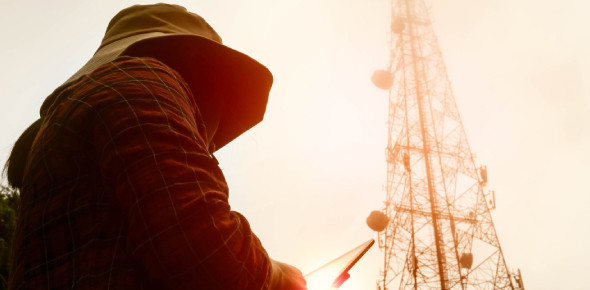# Microwave Engineering Quiz! Exam

19 Questions | Total Attempts: 368Settings.

• 1.
A waveguide section in a microwave circuit acts as:
• A.

LP filter

• B.

All pass filter

• C.

HP filter

• D.

Band stop filter

• 2.
Which of the following devices uses a slow wave structure?
• A.

Klystron two cavity amplifier

• B.

Klystron multicavity amplifier

• C.

Travelling wave tube

• D.

Reflex klystron oscillator

• 3.
A line has Z0 = 300 ∠ 0° Ω. If ZL = 150 ∠ 0° Ω, reflection coefficient is
• A.

0.5

• B.

0.3333

• C.

-0.5

• D.

-0.3333

• 4.
In a directional coupler:
• A.

Isolation (dB) equals coupling plus directivity

• B.

Coupling (dB) equals isolation plus directivity

• C.

Directivity (dB) equals isolation plus coupling

• D.

isolation (dB) equals (coupling) (directivity)

• 5.
The reflection coefficient on a line is 0.2 ∠45°. The SWR is:
• A.

0.8

• B.

1.1

• C.

1.2

• D.

1.5

• 6.
The velocity factor of a transmission line depends on:
• A.

Temperature

• B.

Skin effect

• C.

Relative permittivity of dielectric

• D.

None of the above

• 7.
A frequency at which microwave ovens operate is:
• A.

50 GHz

• B.

8 GHz

• C.

2.45 GHz

• D.

3.5 GHz

• 8.
Which of the following materials are generally preferred for waveguides?
• A.

Cast iron and steel

• B.

Nonmetallic solids including plastic

• C.

High carbon steel and vanadium steel

• D.

Brass and aluminium

• 9.
In a magnetron why do the electrons travel in a cycloidal path?
• A.

The cathode is positive

• B.

Strong field is supplied by the permanent magnet

• C.

The anode is negative

• D.

The cavities are resonant

• 10.
Which of the following is wrong for a magic used to tee?
• A.

E and H arms are decoupled

• B.

Coplanar arms are coupled

• C.

All ports are perfectly matched

• D.

A signal into coplanar arm splits equally between E and H arms

• 11.
Which of the following bands that not comes under Microwave Band?
• A.

C band

• B.

X band

• C.

Ku band

• D.

F band

• 12.
Which of the following is the main advantage of the microwave?
• A.

Highly directive

• B.

Moves at the speed of light

• C.

Greater S/N ratio

• D.

High penetration power

• 13.
_______ is the best medium for handling large microwave power.
• A.

Coaxial line

• B.

Rectangular wave guide

• C.

Strip line

• D.

Copper wires

• 14.
Which of the following is used for amplification of microwave energy?
• A.

Reflex Klystron

• B.

Megnetron

• C.

Travelling wave tube

• D.

Gunn Diode

• 15.
Which of the following microwave tube amplifier uses an axial magnetic field & radial electric field?
• A.

Reflex Klystron

• B.

Coaxial Magnetron

• C.

Travelling Wave Tube

• D.

Crossed field Megnetron

• 16.
The fabrication of microstrip line is done by?
• A.

Etching

• B.

Printed circuit Board technique

• C.

Oxidation

• D.

• 17.
For TE10 mode a rectangular wave guide has dimensions are 7 * 3.5 cm2. its cut off frequency is:
• A.

2.14 GHz

• B.

2.5 GHz

• C.

1.5 GHz

• D.

1.9 GHz

• 18.
For the case of "No Propagation" the propagation constant must be:
• A.

Real

• B.

Imaginary

• C.

Complex

• D.

None of Above

• 19.
The range of VSWR given as:
• A.

0 to 1

• B.

1 to 2

• C.

1 to infinite

• D.

-1 to 1

Related TopicsBack to top